# Physics - Classical Mechanics - Force and Potential Energy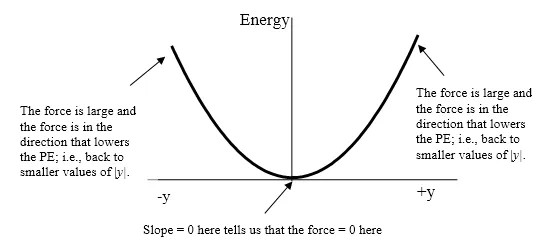[Image 1]

## Introduction

Hey it's a me again @drifter1! After a big pause, today we finally continue with Physics and more specifically the branch "Classical Mechanics" to talk about Force and Potential Energy.

More specifcally, today's topics are:

• Force as the Potential Energy Derivative (one-dimension)
• Force as the Potential Energy Gradient (three-dimensions)

So, without further ado, let's get straight into them!

## Force as the Potential Energy Derivative (one-dimension)

Thinking about the two types of conservative forces and energies that we talked about previously (elastic and gravitational) you might have noticed something!
The gravity force is given by: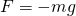whilst the gravitational potential energy is given by: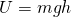In the same way, based on Hooke's law the elastic force is given by: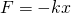whilst the corresponding potential energy is: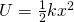Knowing some Mathematical Analysis, you can clearly see that the force can be found using a Differentiation on the potential energy equation. Having both the force and the potential energy in the same dimension and remembering that the work of a force is equal to the negative of the change in potential energy (W = -ΔU), we can easily talk about infinitesimal changes in position (dx).

So, in the end the force in one dimension is given by: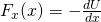### Example:

The electric potential energy of a specific system is given by: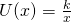Calculating the electric force is pretty simple and looks like this: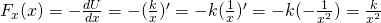## Force as the Potential Energy Gradient (three-dimensions)

Expanding our analysis into 2, 3 or even more dimension is quite easy! The force along the other dimensions (y and z) can be found using a differentiation on the equation using the variable of that dimension(dy and dz). This clearly gets us into partial differentiation, which is based on exactly that. So, the 3 equations for F look like this:Using the unit vectors for each dimension (i, j and k) we can write: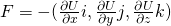In the end, the force is equal to the negative gradient of the potential energy: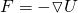### Example:

Consider the following Potential energy equation: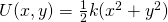Let's calculate the applied force equation in vector form!
Using partial differentiation we calculate the equation for each of the two axes:So, the total force is: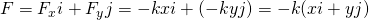## RESOURCES:

### References

1. https://phys.libretexts.org/Courses/University_of_California_Davis/UCD%3A_Physics_7A/2%3A_Applying_Models_to_Mechanical_Phenomena/2.5%3A_Force_and_Potential_Energy
2. http://hyperphysics.phy-astr.gsu.edu/hbase/pegrav.html

### Images

1. https://phys.libretexts.org/Courses/University_of_California_Davis/UCD%3A_Physics_7A/2%3A_Applying_Models_to_Mechanical_Phenomena/2.5%3A_Force_and_Potential_Energy

## Final words | Next up

This is actually it for today's post! Next time we will continue with even more stuff around Potential Energy.

See ya!Keep on drifting!

H2
H3
H4
3 columns
2 columns
1 column
1 Comment